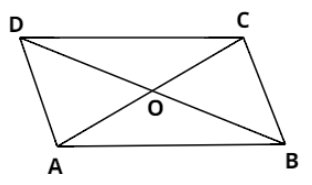Courses
Courses for Kids
Free study material
Free LIVE classes
MoreLIVE
Join Vedantu’s FREE Mastercalss

# Given a parallelogram ABCD. Complete each statement along with the definition used:$(i)$ $AD =$ $(ii)$ $\angle DCB =$ $(iii)$ $OC =$ $(iv)$ $m\angle DAB + m\angle CDA =$Verified
360.9k+ views
Hint: Use the properties of parallelogram: Opposite sides are parallel and equal, opposite angles are equal and sum of adjacent angles is ${180^ \circ }$.

Given, ACBCD is a parallelogram with O as the point of intersection of its diagonals AC and BD.
$(i)$ We know that in parallelogram, lengths of opposite sides are equal. Hence, we can conclude that:
$\Rightarrow AD = BC$
$(ii)$ We know that in parallelogram, measure of opposite angles is equal. Hence, we can conclude that:
$\Rightarrow \angle DCB = \angle DAB$
$(iii)$ We know that in parallelogram, diagonals bisect each other. Hence, we can conclude that:
$\Rightarrow OC = OA$
$(iv)$ We know that in parallelogram, adjacent angles are supplementary (i.e. their sum is ${180^ \circ }$). Hence, we can conclude that:
$\Rightarrow m\angle DAB + m\angle CDA = {180^ \circ }$

Note: In parallelogram, although the diagonals bisect each other but their lengths are not equal.
In the above parallelogram, $AC \ne BD$.
Last updated date: 25th Sep 2023
Total views: 360.9k
Views today: 8.60k